# Balbharati solutions for Mathematics 2 Geometry 10th Standard SSC Maharashtra State Board chapter 3 - Circle [Latest edition]

#### Chapters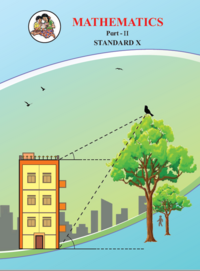## Chapter 3: Circle

Practice Set 3.1Practice Set 3.2Practice Set 3.3Practice Set 3.4Practice Set 3.5Problem Set 3
Practice Set 3.1 [Page 55]

### Balbharati solutions for Mathematics 2 Geometry 10th Standard SSC Maharashtra State Board Chapter 3 Circle Practice Set 3.1 [Page 55]

Practice Set 3.1 | Q 1 | Page 55

In the adjoining figure the radius of a circle with centre C is 6 cm, line AB is a tangent at A. Answer the following questions.
(1) What is the measure of ∠CAB ? Why ?
(2) What is the distance of point C from line AB? Why ?
(3) d(A,B) = 6 cm, find d(B,C).
(4) What is the measure of ∠ABC ? Why ?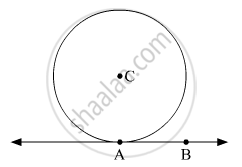Practice Set 3.1 | Q 2 | Page 55

In the adjoining figure, O is the centre of the circle. From point R, seg RM and seg RN are tangent segments touching the circle at M and N. If (OR) = 10 cm and radius of the circle = 5 cm, then
(1) What is the length of each tangent segment ?
(2) What is the measure of ∠MRO ?
(3) What is the measure of ∠ MRN ?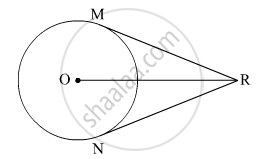Practice Set 3.1 | Q 3 | Page 55

Seg RM and seg RN are tangent segments of a circle with centre O. Prove that seg OR bisects ∠MRN as well as ∠MON with the help of activity.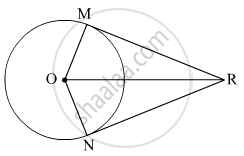Practice Set 3.1 | Q 4 | Page 55

What is the distance between two parallel tangents of a circle having radius 4.5 cm ? Justify your answer.

Practice Set 3.2 [Page 58]

### Balbharati solutions for Mathematics 2 Geometry 10th Standard SSC Maharashtra State Board Chapter 3 Circle Practice Set 3.2 [Page 58]

Practice Set 3.2 | Q 1 | Page 58

Two circles having radii 3.5 cm and 4.8 cm touch each other internally. Find the distance between their centres.

Practice Set 3.2 | Q 2 | Page 58

Four alternative answers for the following question is given. Choose the correct alternative.

Two circles of radii 5.5 cm and 4.2 cm touch each other externally. Find the distance between their centres

• 9.7

• 1.3

• 2.6

• 4.6

Practice Set 3.2 | Q 3 | Page 58

If radii of two circles are 4 cm and 2.8 cm. Draw figure of these circles touching each other – (i) externally (ii) internally.

Practice Set 3.2 | Q 4 | Page 58

In the given figure, the circles with centres P and Q touch each other at R. A line passing through R meets the circles at A and B respectively. Prove that – (1) seg AP || seg BQ,
(2) ∆APR ~ ∆RQB, and
(3) Find ∠ RQB if ∠ PAR = 35°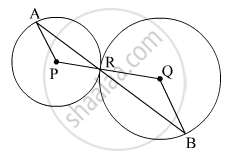Practice Set 3.2 | Q 5 | Page 58

In the given figure, the circles with centres A and B touch each other at E. Line l is a common tangent which touches the circles at C and D respectively. Find the length of seg CD if the radii of the circles are 4 cm, 6 cm.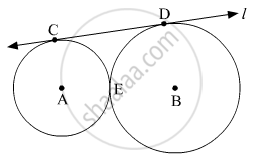Practice Set 3.3 [Pages 63 - 64]

### Balbharati solutions for Mathematics 2 Geometry 10th Standard SSC Maharashtra State Board Chapter 3 Circle Practice Set 3.3 [Pages 63 - 64]

Practice Set 3.3 | Q 1 | Page 63

In the given figure, points G, D, E, F are concyclic points of a circle with centre C.
∠ ECF = 70°, m(arc DGF) = 200° find m(arc DE) and m(arc DEF).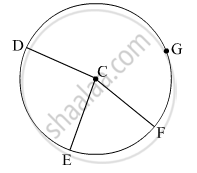Practice Set 3.3 | Q 2 | Page 64

In the given figure, ∆QRS is an equilateral triangle. Prove that,
(1) arc RS ≅ arc QS ≅ arc QR
(2) m(arc QRS) = 240°.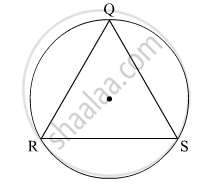Practice Set 3.3 | Q 3 | Page 64

In the given figure, chord AB ≅ chord CD, Prove that, arc AC ≅ arc BD.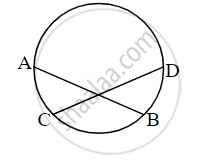Practice Set 3.4 [Pages 73 - 74]

### Balbharati solutions for Mathematics 2 Geometry 10th Standard SSC Maharashtra State Board Chapter 3 Circle Practice Set 3.4 [Pages 73 - 74]

Practice Set 3.4 | Q 1 | Page 73

In the given figure, in a circle with centre O, length of chord AB is equal to the radius of the circle. Find measure of each of the following.
(1) ∠ AOB (2)∠ ACB
(3) arc AB (4) arc ACB.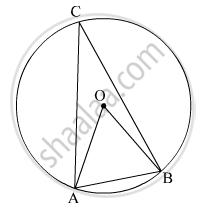Practice Set 3.4 | Q 2 | Page 73

In the given figure, ▢PQRS is cyclic. side PQ ≅ side RQ. ∠ PSR = 110°, Find–
(1) measure of ∠ PQR
(2) m(arc PQR)
(3) m(arc QR)
(4) measure of ∠ PRQ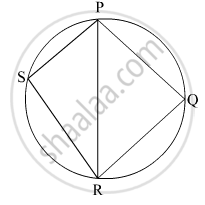Practice Set 3.4 | Q 3 | Page 73

▢MRPN is cyclic, ∠ R = (5x – 13)°, ∠ N = (4x + 4)°. Find measures of ∠ R and ∠ N.

Practice Set 3.4 | Q 4 | Page 73

In the given figure, seg RS is a diameter of the circle with centre O. Point T lies in the exterior of the circle. Prove that ∠ RTS is an acute angle.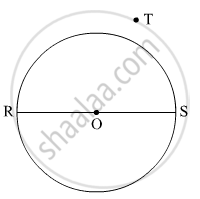Practice Set 3.4 | Q 5 | Page 73

Prove that, any rectangle is a cyclic quadrilateral

Practice Set 3.4 | Q 6 | Page 74

In the given figure, altitudes YZ and XT of ∆WXY intersect at P. Prove that,
(1) ▢WZPT is cyclic.
(2) Points X, Z, T, Y are concyclic.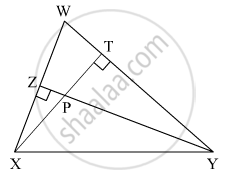Practice Set 3.4 | Q 7 | Page 74

In the given figure, m(arc NS) = 125°, m(arc EF) = 37°, find the measure ∠ NMS.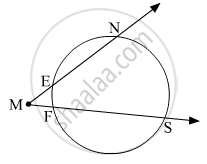Practice Set 3.4 | Q 8 | Page 74

In the given figure, chords AC and DE intersect at B. If ∠ ABE = 108°, m(arc AE) = 95°, find m(arc DC).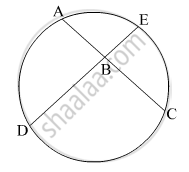Practice Set 3.5 [Page 82]

### Balbharati solutions for Mathematics 2 Geometry 10th Standard SSC Maharashtra State Board Chapter 3 Circle Practice Set 3.5 [Page 82]

Practice Set 3.5 | Q 1 | Page 82

In the given figure, ray PQ touches the circle at point Q. PQ = 12, PR = 8, find PS and RS.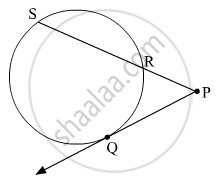Practice Set 3.5 | Q 2 | Page 82

In the given figure, chord MN and chord RS intersect at point D.
(1) If RD = 15, DS = 4, MD = 8 find DN
(2) If RS = 18, MD = 9, DN = 8 find DS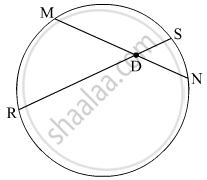Practice Set 3.5 | Q 3 | Page 82

In the given figure, O is the centre of the circle and B is a point of contact. seg OE ⊥ seg AD, AB = 12, AC = 8, find
(1) AD (2) DC (3) DE.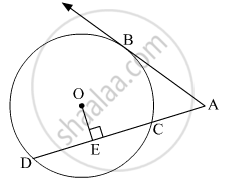Practice Set 3.5 | Q 4 | Page 82

In the given figure, if PQ = 6, QR = 10, PS = 8 find TS.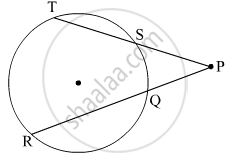Practice Set 3.5 | Q 5 | Page 82

In the given figure, seg EF is a diameter and seg DF is a tangent segment. The radius of the circle is r. Prove that, DE × GE = 4r2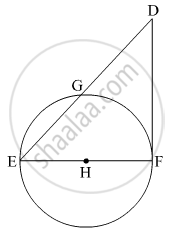Problem Set 3 [Pages 82 - 90]

### Balbharati solutions for Mathematics 2 Geometry 10th Standard SSC Maharashtra State Board Chapter 3 Circle Problem Set 3 [Pages 82 - 90]

Problem Set 3 | Q 1.01 | Page 83

Four alternative answers for the following question is given. Choose the correct alternative.
Two circles of radii 5.5 cm and 3.3 cm respectively touch each other. What is the distance between their centers ?

• 4.4 cm

• 8.8 cm

• 2.2 cm

• 8.8 or 2.2 cm

Problem Set 3 | Q 1.02 | Page 82

Four alternative answers for the following question is given. Choose the correct alternative.

Two circles intersect each other such that each circle passes through the centre of the other. If the distance between their centres is 12, what is the radius of each circle?

• 6 cm

• 12 cm

• 24 cm

• can’t say

Problem Set 3 | Q 1.03 | Page 83

Four alternative answers for the following question is given. Choose the correct alternative.

A circle touches all sides of a parallelogram. So the parallelogram must be a, ______

• rectangle

• rhombus

• square

• trapezium

Problem Set 3 | Q 1.04 | Page 83

Four alternative answers for the following question is given. Choose the correct alternative.
Length of a tangent segment drawn from a point which is at a distance 12.5 cm from the centre of a circle is 12 cm, find the diameter of the circle.

• 25 cm

• 24 cm

• 7 cm

• 14 cm

Problem Set 3 | Q 1.05 | Page 83

Four alternative answers for the following question is given. Choose the correct alternative.
If two circles are touching externally, how many common tangents of them can be drawn?

• One

• Two

• Three

• Four

Problem Set 3 | Q 1.06 | Page 83

Four alternative answers for the following question is given. Choose the correct alternative.

∠ACB is inscribed in arc ACB of a circle with centre O. If ∠ACB = 65°, find m(arc ACB).

• 65°

• 130°

• 295°

• 230°

Problem Set 3 | Q 1.07 | Page 83

Four alternative answers for the following question is given. Choose the correct alternative.
Chords AB and CD of a circle intersect inside the circle at point E. If AE = 5.6, EB = 10, CE = 8, find ED.

• 7

• 8

• 11.2

• 9

Problem Set 3 | Q 1.08 | Page 83

Four alternative answers for the following question is given. Choose the correct alternative

In a cyclic ▢ABCD, twice the measure of ∠A is thrice the measure of ∠C. Find the measure of ∠C?

• 36°

• 72°

• 90°

• 108°

Problem Set 3 | Q 1.09 | Page 83

Four alternative answers for the following question is given. Choose the correct alternative.

Points A, B, C are on a circle, such that m(arc AB) = m(arc BC) = 120°. No point, except point B, is common to the arcs. Which is the type of ∆ABC?

• Equilateral triangle

• Scalene triangle

• Right angled triangle

• Isosceles triangle

Problem Set 3 | Q 1.1 | Page 83

Four alternative answers for the following question is given. Choose the correct alternative.

Seg XZ is a diameter of a circle. Point Y lies in its interior. How many of the following statements are true ? (i) It is not possible that ∠XYZ is an acute angle. (ii) ∠XYZ can’t be a right angle. (iii) ∠XYZ is an obtuse angle. (iv) Can’t make a definite statement for measure of ∠XYZ.

• Only one

• Only two

• Only three

• All

Problem Set 3 | Q 2 | Page 84

Line l touches a circle with centre O at point P. If radius of the circle is 9 cm, answer the following.
(1) What is d(O, P) = ? Why ?
(2) If d(O, Q) = 8 cm, where does the point Q lie ?
(3) If d(OQ) = 15 cm, How many locations of point Q are line on line l? At what distance will each of them be from point P?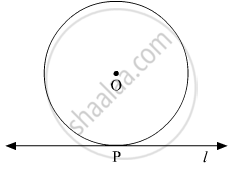Problem Set 3 | Q 3 | Page 84

In the given figure, M is the centre of the circle and seg KL is a tangent segment.
If MK = 12, KL = $6\sqrt{3}$ then find –
(2) Measures of ∠K and ∠M.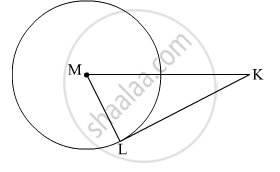Problem Set 3 | Q 4 | Page 84

In the given figure, O is the centre of the circle. Seg AB, seg AC are tangent segments. Radius of the circle is r and l(AB) = r, Prove that ▢ABOC is a square.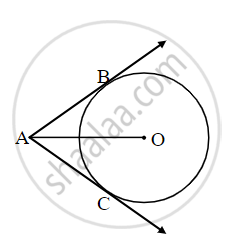Proof: Draw segment OB and OC.

l(AB) = r      ......[Given] (I)

AB = AC    ......[square] (II)

But OB = OC = r    ......[square] (III)

From (i), (ii) and (iii)

AB = square = OB = OC = r

∴ Quadrilateral ABOC is square

Similarly, ∠OBA = square      ......[Tangent Theorem]

If one angle of square is right angle, then it is a square.

∴ Quadrilateral ABOC is a square.

Problem Set 3 | Q 5 | Page 85

In the given figure, ▢ABCD is a parallelogram. It circumscribes the circle with centre T. Point E, F, G, H are touching points. If AE = 4.5, EB = 5.5, find AD.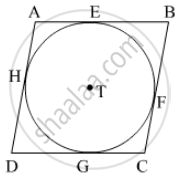Problem Set 3 | Q 6 | Page 85

In the given figure, circle with centre M touches the circle with centre N at point T. Radius RM touches the smaller circle at S. Radii of circles are 9 cm and 2.5 cm. Find the answers to the following questions hence find the ratio MS:SR.
(1) Find the length of segment MT
(2) Find the length of seg MN
(3) Find the measure of ∠NSM.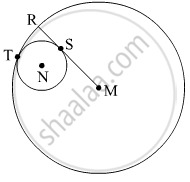Problem Set 3 | Q 7 | Page 85

In the adjoining figure circles with centres X and Y touch each other at point Z. A secant passing through Z intersects the circles at points A and B respectively. Prove that, radius XA || radius YB. Fill in the blanks and complete the proof.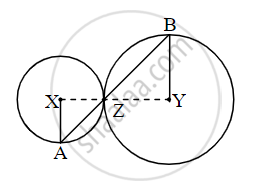Construction: Draw segments XZ and YZ.

Proof:

By theorem of touching circles, points X, Z, Y are square.
∴ ∠XZA ≅ square                     ...(opposite angles)
Let ∠XZA = ∠BZY = a       ...(I)
Now, seg XA ≅ seg XZ      ...[Radii of the same circle]
∴∠XAZ = square = a                ...[isosceles triangle theorem](II)
Similarly,

seg YB ≅ seg YZ            ...[Radii of the same circle]
∴∠BZY = square = a   ...[isosceles triangle theorem](III)
∴ from (I), (II), (III),
∠XAZ = square
∴ radius XA || radius YZ     ...[square]

Problem Set 3 | Q 8 | Page 86

In the given figure, circles with centres X and Y touch internally at point Z . Seg BZ is a chord of bigger circle and it itersects smaller circle at point A. Prove that, seg AX || seg BY.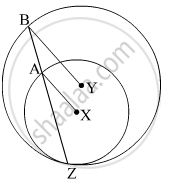Problem Set 3 | Q 9 | Page 86

In the figure, line l touches the circle with center O at point P. Q is the midpoint of radius OP. RS is a chord through Q such that chords RS || line l. If RS = 12, find the radius of the circle.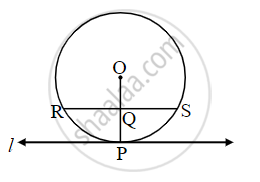Problem Set 3 | Q 10 | Page 86

In the given figure, seg AB is a diameter of a circle with centre C. Line PQ is a tangent, which touches the circle at point T. seg AP ⊥ line PQ and seg BQ ⊥ line PQ. Prove that, seg CP ≅ seg CQ.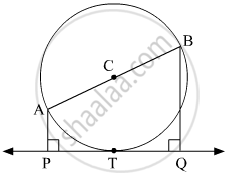Problem Set 3 | Q 11 | Page 86

Draw circles with centres A, B and C each of radius 3 cm, such that each circle touches the other two circles.

Problem Set 3 | Q 12 | Page 86

Prove that any three points on a circle cannot be collinear.

Problem Set 3 | Q 13 | Page 87

In the given figure, line PR touches the circle at point Q. Answer the following questions with the help of the figure.
(1) What is the sum of ∠ TAQ and ∠ TSQ ?
(2) Find the angles which are congruent to ∠ AQP.
(3) Which angles are congruent to ∠ QTS ?
(4) ∠TAS = 65°, find the measure of ∠TQS and arc TS.
(5) If ∠AQP = 42°and ∠SQR = 58° find measure of ∠ATS.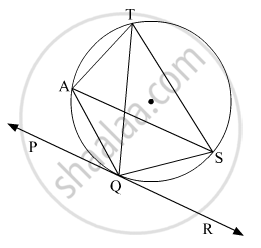Problem Set 3 | Q 14 | Page 87

In the given figure, O is the centre of a circle, chord PQ ≅ chord RS If ∠ POR = 70° and (arc RS) = 80°, find –
(1) m(arc PR)
(2) m(arc QS)
(3) m(arc QSR)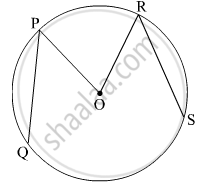Problem Set 3 | Q 15 | Page 87

In the given figure, m(arc WY) = 44°, m(arc ZX) = 68°, then
(1) Find the measure of ∠ ZTX.
(2) If WT = 4.8, TX = 8.0,
YT = 6.4, find TZ.
(3) If WX = 25, YT = 8,
YZ = 26, find WT.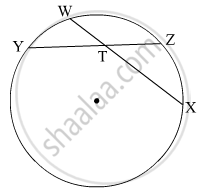Problem Set 3 | Q 16 | Page 88

In the given figure
(1) m(arc CE) = 54°, m(arc BD) = 23°, find measure of ∠CAE.
(2) If AB = 4.2, BC = 5.4, AE = 12.0, find AD
(3) If AB = 3.6, AC = 9.0, AD = 5.4, find AE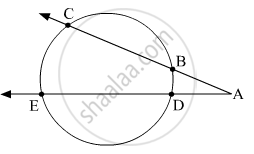Problem Set 3 | Q 17 | Page 88

In the given figure, chord EF || chord GH. Prove that, chord EG ≅ chord FH. Fill in the blanks and write the proof.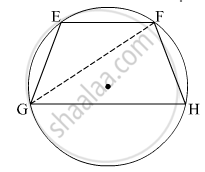Problem Set 3 | Q 18 | Page 88

In the given figure, P is the point of contact.
(1) If m(arc PR) = 140°, ∠ POR = 36°, find m(arc PQ)
(2) If OP = 7.2, OQ = 3.2, find OR and QR
(3) If OP = 7.2, OR = 16.2, find QR.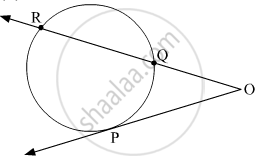Problem Set 3 | Q 19 | Page 88

In the given figure, circles with centres C and D touch internally at point E. D lies on the inner circle. Chord EB of the outer circle intersects inner circle at point A. Prove that, seg EA ≅ seg AB.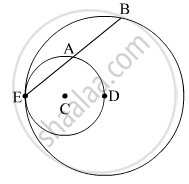Problem Set 3 | Q 20 | Page 89

In the given figure, seg AB is a diameter of a circle with centre O. The bisector of ∠ ACB intersects the circle at point D. Prove that, seg AD ≅ seg BD. Complete the following proof by filling in the blanks.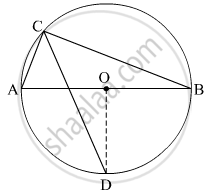Problem Set 3 | Q 21 | Page 89

In the given figure, seg MN is a chord of a circle with centre O. MN = 25, L is a point on chord MN such that ML = 9 and d(O,L) = 5. Find the radius of the circle.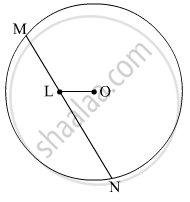Problem Set 3 | Q 22 | Page 89

In the given figure, two circles intersect each other at points S and R. Their common tangent PQ touches the circle at points P, Q.
Prove that, ∠ PRQ + ∠ PSQ = 180°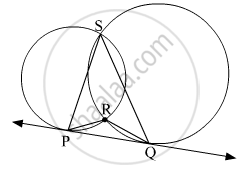Problem Set 3 | Q 23 | Page 90

In the given figure, two circles intersect at points M and N. Secants drawn through M and N intersect the circles at points R, S and P, Q respectively. Prove that : seg SQ || seg RP.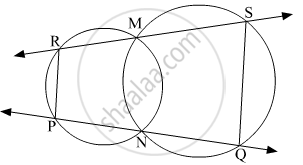Problem Set 3 | Q 24 | Page 90

In the given figure, two circles intersect each other at points A and E. Their common secant through E intersects the circles at points B and D. The tangents of the circles at points B and D intersect each other at point C. Prove that ▢ABCD is cyclic.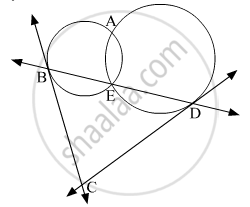Problem Set 3 | Q 25 | Page 90

In the given figure, seg AD ⊥ side BC, seg BE ⊥ side AC, seg CF ⊥ side AB. Ponit O is the orthocentre. Prove that , point O is the incentre of ∆DEF.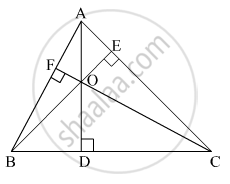## Chapter 3: Circle

Practice Set 3.1Practice Set 3.2Practice Set 3.3Practice Set 3.4Practice Set 3.5Problem Set 3## Balbharati solutions for Mathematics 2 Geometry 10th Standard SSC Maharashtra State Board chapter 3 - Circle

Balbharati solutions for Mathematics 2 Geometry 10th Standard SSC Maharashtra State Board chapter 3 (Circle) include all questions with solution and detail explanation. This will clear students doubts about any question and improve application skills while preparing for board exams. The detailed, step-by-step solutions will help you understand the concepts better and clear your confusions, if any. Shaalaa.com has the Maharashtra State Board Mathematics 2 Geometry 10th Standard SSC Maharashtra State Board solutions in a manner that help students grasp basic concepts better and faster.

Further, we at Shaalaa.com provide such solutions so that students can prepare for written exams. Balbharati textbook solutions can be a core help for self-study and acts as a perfect self-help guidance for students.

Concepts covered in Mathematics 2 Geometry 10th Standard SSC Maharashtra State Board chapter 3 Circle are Theorem - Converse of Tangent at Any Point to the Circle is Perpendicular to the Radius, Number of Tangents from a Point on a Circle, Inscribed Angle Theorem, Intercepted Arc, Inscribed Angle, Property of Sum of Measures of Arcs, Tangent Segment Theorem, Converse of Tangent Theorem, Circles Passing Through One, Two, Three Points, Theorem of External Division of Chords, Theorem of Internal Division of Chords, Converse of Theorem of the Angle Between Tangent and Secant, Theorem of Angle Between Tangent and Secant, Converse: If a pair of opposite angles of a quadrilateral is supplementary, then the quadrilateral is cyclic., Corollary of Cyclic Quadrilateral Theorem, Theorem: Opposite angles of a cyclic quadrilateral are supplementary., Corollaries of Inscribed Angle Theorem, Angle Subtended by the Arc to the Point on the Circle, Angle Subtended by the Arc to the Centre, Introduction to an Arc, Touching Circles, Number of Tangents from a Point on a Circle, Tangent to a Circle, Tangents and Its Properties, Tangent Properties - If Two Circles Touch, the Point of Contact Lies on the Straight Line Joining Their Centers, Cyclic Properties, Tangent - Secant Theorem, Cyclic Quadrilateral, Concept of Circle - Centre, Radius, Diameter, Arc, Sector, Chord, Segment, Semicircle, Circumference, Interior and Exterior, Concentric Circles.

Using Balbharati 10th Standard Board Exam solutions Circle exercise by students are an easy way to prepare for the exams, as they involve solutions arranged chapter-wise also page wise. The questions involved in Balbharati Solutions are important questions that can be asked in the final exam. Maximum students of Maharashtra State Board 10th Standard Board Exam prefer Balbharati Textbook Solutions to score more in exam.

Get the free view of chapter 3 Circle 10th Standard Board Exam extra questions for Mathematics 2 Geometry 10th Standard SSC Maharashtra State Board and can use Shaalaa.com to keep it handy for your exam preparation